Ask the virtual scientist### Types of atmospheres

PSG handles two types of atmospheres: hydrostatic equilibrium (typical for planets) and expanding coma (typical of comets and small bodies). In both cases, a set of three parameters can be used to describe the overall structure of the atmosphere: temperature (K), mean molecular weight and surface pressure (for equilibrated atmospheres) or production rate (for expanding ones).

PSG permits providing detailed vertical information of molecular abundances and temperatures, and atmospheric templates (vertical profiles of temperature and abundances) are available for the main atmospheres (Venus, Earth, Mars, Titan, Neptune, Uranus), while general atmospheric and surface parameters are available for the other bodies. For expanding atmospheres, PSG assumes isotropic outflow, constant expanding velocity (following vexp = 0.8 Rh-0.5 km/s) and a dust outgassing model.

The approximate brightness for the object (and for the parent star in the case of exoplanets) is displayed in the surface section, which is computed based on the object size, albedo, distances and stellar temperature. For cometary coma, three brightness metrics are calculated and displayed next to the production rate field: the expected visual magnitude (mv), the infrared Figure-Of-Merit (FOMIR), and the radio Figure-Of-Merit (FOMRadio). These parameters are calculated based on the heliocentric distance (RH, [AU]), the geocentric distance (Delta, [AU]), and the gas production rate (Q, [mol/s]), employing these approximations: mv = (30.675 - log(Q))/0.2453 + 5⋅log(Delta) [Jorda et al. 2008]; FOMIR = Q⋅1E-29/(Delta⋅RH2); FOMRadio = Q⋅1E-28/Delta; and where Q = QAU/(RH2).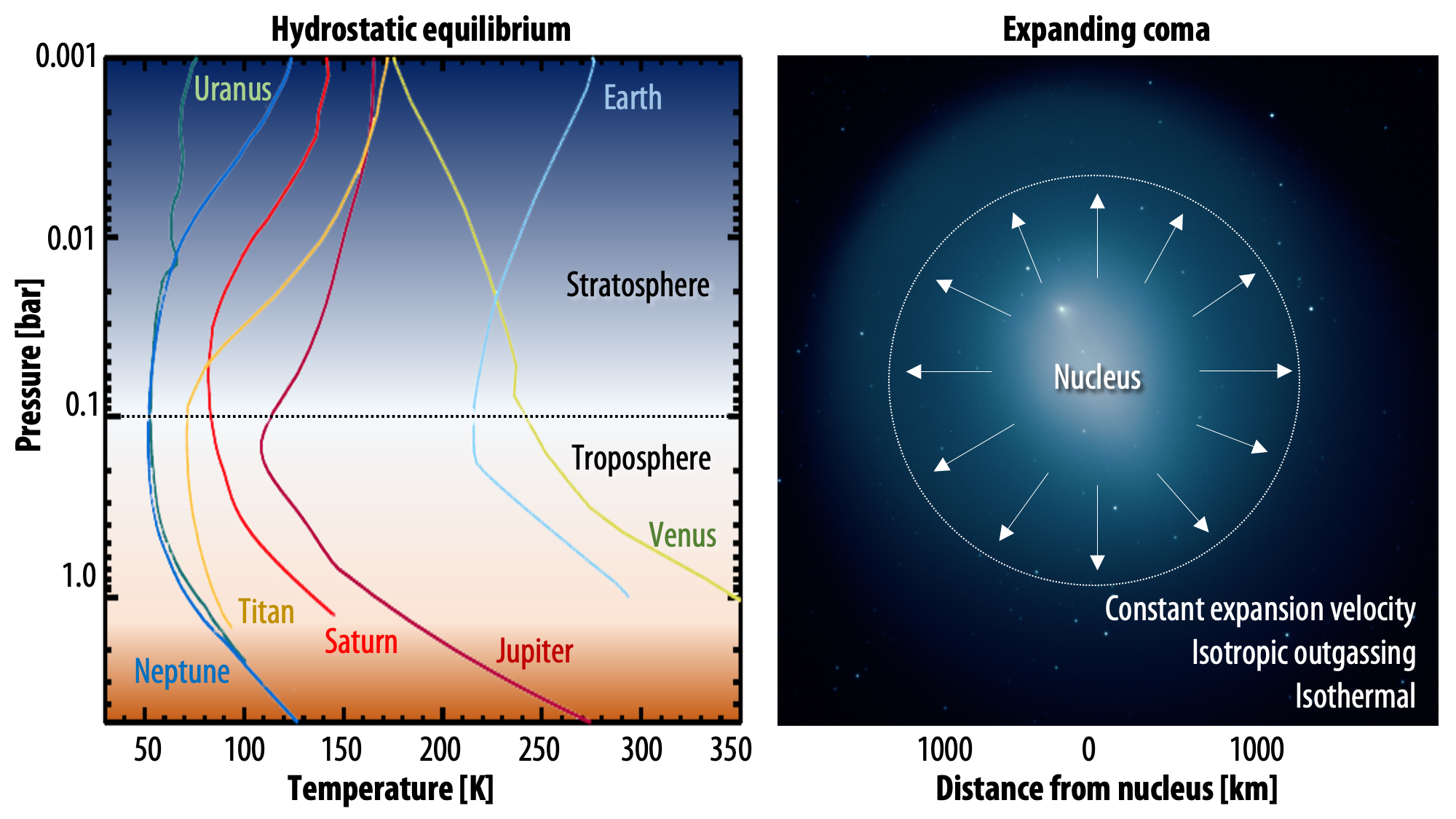Types of atmospheres: the user can select between two types of atmospheres: hydrostatic equilibrium (typical for planets, profiles adapted from Robinson & Catling 2014) and expanding coma (typical of comets and small bodies). For the main planets, vertical profiles are available, while the user can also load any arbitrary vertical strucutre. For expanding atmospheres, PSG assumes isotropic outgassing, a constant temperature across the coma, and an outgassing velocity established by the heliocentric distance.

### Defining the profiles and abundances

Two important steps are addressed in this section: 1) the structure and composition of the atmosphere/surface, 2) the databases that are being used for each component. PSG ingests a broad range of spectroscopic information, with the following types:

0:REFLReflectance: the reflecting properities of the material are described as a scaling factor (0-1).
1:OPTCOptical constants: the spectroscopy is tabulated as n-k, where n is the refractive index and k is the extinction coefficient.
2:ALPHAlpha parameter: the α index indicates the extinction coefficient per slab width.
3:XSECCross sections: the molecular absorptions are described as a table of cross-sections [cm2/molecule].
4:SCALScattering function (Legendre): the scattering function is described as a summation of Legendre polynomials.
5:SCATScattering function (Henyey-Greenstein): the scattering function is defined as a H-G function.
6:LBLNLine-by-line database: each line is described individually, requiring expensive line-by-line RT calculations.
7:CKTBCorrelated-k tables: pre-computed corr-k opacity tables are available in PSG for efficient RT calculations.
8:MASSMass spectrometry library: mass spectral fragmentation pattern assuming electron ionization.

The user can provide an arbitrary profile of P/T and of molecular abundances by selecting "File Template". The format of this file is a text file with all the entries as in the configuration file for the sections "ATMOSPHERE" and "SURFACE" (download example). The pressures are provided in 'bars', temperatures in 'K' and abundances in volume mixing ratio - [molecules / molecules] for gases (e.g., water vapor, methane) and [kg / kg] for hazes (e.g., water ice clouds, methane ice). Particle sizes in [m] are provided by adding the keyword '_size'. The base (molecules or kg) is always wet air (all gases, including water vapor) without hazes. For Earth, the standard profiles (US-Standard, Tropical, mid-latitude-winter, midlatitude-summer, subarctic-summer, subarctic-winter) are available by selecting "Template", while for Mars an array of climatological templates computed using the LMD General Circulation Model (Millour et al. 2008) are available.

For exoplanets, PSG will select from a list of pre-computed templates (considering the planet's mass and density), and will compute P/T profiles for giants based on a non-gray analytical model (Parmentier et al. 2014, A&A, Vol. 562, A133) according to the planet's equilibrium temperature (based on distance to star, stellar radius and stellar temperature) and the planetary gravity. Abundances are computed layer-by-layer considering the equation-of-states (EOS) computed by Kempton et al. (PASP, Vol. 129, Issue 974, pp. 044402, 2017; Mbarek & Kempton, ApJ, Vol. 827, Issue 2, id. 121, pp. 10, 2016) for the T-P grid of 100 to 3000 K and 1000 to 1E-9 bar.

When no vertical profile is provided, the temperature (T) is assumed to be constant across the atmosphere, and the pressure (P) decreases with altitude (z) following the scale-height: P = Psurf exp(-zg/RT), where g is the gravity (defined in the object section) and R is the gas constant (8.3144598 J / K / mol).

In order to change the abundance of gases (or their isotopologues), first select the gas in the left column (press "Search" to search for available species within the catalogues), then select the isotopologue / linelist in the "Type" section. For instance, the HITRAN database is described by "HIT" (first number is mol ID, second is isotopic numer), the JPL Molecular Spectroscopy database with "JPL", the Cologne Database of Molecular Spectroscopy by "CDMS", and the GSFC Fluorescence database by "GSFC".

Scattering aerosols: most atmospheres are a mix of gases and of aerosols (dust/ice/clouds/hazes), with the latter scattering the light in a complex pattern depending on the viewing angle, aerosol size/shape and composition. Aerosols abundances can be provided in terms of aerosol mass content (kg/kg), a scaling factor (scl) of the provided vertical profile or as a fixed value across alitude (%, ppm, ppb, ppt, kg/m2 for the column). When an aerosol profile is available, PSG will employ this vertical distribution, but will scale the column to match the defined total column (kg/m2).

### Molecular/atomic databases (8727 species)

The key parameter to be entered in the "Atmosphere" section is the definition of the molecular species and the corresponding linelist to be used for these molecules. HITRAN is generally very complete (for IR, optical and UV at low temperatures) for most typical planetary atmosphere and has become the main repository of line information. At radio wavelengths, the JPL Molecular Spectroscopy and Cologne Database of Molecular Spectroscopy (CDMS) are generally more complete and have a better description of the rotational spectrum of complex molecules. NASA-Goddard currently holds the main repository for non-LTE fluorescence linelists, suitable when synthesizing cometary spectra in the UV/optical/IR range. For mass spectrometry, PSG accesses the publicly available NIST Chemistry WebBook (SRD 69) database.

 Molecule  Type### Opacity generator

 Molecule  Type Frequency/wavelength range: um nm mm Angstrom cm-1 MHz GHz kHz    Resolution: Resolving power um nm mm Angstrom cm-1 MHz GHz kHz Pressure: bar Pascal kbar mbar ubar at atm Torr psi Temperature [K]:   Threshold (for linelist):%%%%% The collissional and excitation environment will be based on these parameters and the availability of spectroscopic information (see databases above).

### Scattering aerosols (105 species)

The presence of aerosol particles (dust/ice/clouds/hazes) in a planetary atmosphere has a significant impact on the intensity and morphology of planetary spectra, and can severely hinder the ability to infer atmospheric properties such as abundances from gaseous absorption signatures and atmospheric temperatures (e.g., Sing et al. 2013; Vasquez et al. 2013a; 2013b). In addition, without explicitly including the radiative effects of aerosols (including their particular microphysical properties), the inconsistencies between the predicted spectra and the subsequently inferred equation of state are likely to be considerable, as has been demonstrated for the well-studied case of Mars (e.g., Madeleine et al. 2011; 2012).

The general approach of the community has involved the coupling of a highly developed molecular transmission code (i.e., LBLRTM, GENLN3) with a multiple scattering radiative transfer algorithm (i.e., DISORT, Stamnes et al. 1988; Lin et al. 2015) such as has been done by Turner and collaborators with LBLDIS (Turner 2005). However, while these codes are publicly available and include documentation by the authors, their use as a tool has generally been limited to radiative transfer specialists and the availability of non-trivial computational resources. In PSG, we provide this capability for specific set of modes that balance spectral resolution and range with computation efficiency.

A treatment of multiple scattering from atmospheric aerosols is enabled within PSG using the discrete ordinates method (e.g., Goody and Yung, 1989; Thomas and Stamnes, 1999; Smith et al., 2013). The radiation field is approximated by a discrete number of streams distributed in angle with respect to the plane-parallel normal. The number of stream pairs (pairs of corresponding upward and downward radiation streams) can be set as high as necessary to accurately model the angular dependence of the aerosol scattering phase function while maintaining computational feasibility. The “two-stream approximation” often used when modeling planetary atmospheres is an example of the discrete ordinates method using one stream pair.

The angular dependence of the scattering phase function for a particular aerosol is described in terms of an expansion in terms of Legendre Polynomials, typically with the number of expansion terms equal to the number of stream pairs. As implemented in PSG, the Legendre expansion coefficients are pre-computed using an assumed particle size distribution for each available aerosol type and using either Mie scattering (e.g., Wiscombe, 1980) or T-matrix (e.g., Mishchenko et al., 1996) codes as specified in the associated information files for each aerosol type. The underlying indices of refraction for aerosols are empirically derived from spacecraft observations in the case of Mars dust and water ice aerosols (Wolff et al., 2009), or from the HRI (HITRAN Refractory Index, Massie and Hervig 2013 and references therein) database. For the case of the HRI constants, we calculate scattering coefficients employing a Mie implementation (Bohren and Huffman 1983) that derives Henyey-Greenstein scattering g-factors. Internally, PSG converts these g-factors into Legendre expansion coefficients in order to be ingested by the radiative-transfer suite.

The discrete ordinates formulation computes the diffuse radiation field for a plane-parallel atmosphere. When spherical geometry is important (e.g., limb geometry observations), the pseudo-spherical approximation (e.g., Spurr, 2002; Thomas and Stamnes, 1999) is used for computational efficiency. In this scheme, the source functions computed using the diffuse field from the discrete ordinates plane-parallel geometry are integrated along an equivalent curved path through the model layers. This curved path is defined by computing the correct emission angle for the path at the boundary of each layer. The pseudo-spherical approximation is accurate over a wide range of conditions and is orders of magnitude faster than an “exact” Monte Carlo code (Smith et al., 2013).

### Molecular (Rayleigh) and aerosols (Mie) scattering

The two main processes dominating scattering in an atmosphere can be separared into molecular (Rayleigh) scattering and aerosols (Mie) scattering. Molecular scattering results from the electric polarizability by molecules, which are much much smaller than the wavelength of the radiation. The amount of scattering is inversely proportional to the fourth power of the wavelength, so its effects is prominently at short wavelengths (lower than 1 micron). Aerosols scattering can be modelled employing Mie theory (Rayleigh scattering can be also described with Mie theory), and therefore this type of scattering by small particles is called Mie scattering. Molecular scattering tends to be more isotropic, while aerosols scattering is more promiment at wavelengths comparable to the size of the particles, and tends to have a very directed phase function, leading to notable asymmetries in the observed fluxes with respect to phase angle. In the examples below, we show synthetic all-sky images computed with PSG considering molecular scattering and aerosols scattering for several planets (e.g., Earth, Mars, Titan, Uranus) and aerosol content.

 Earth's clear Earth's hazy atmosphere Earth overcast weather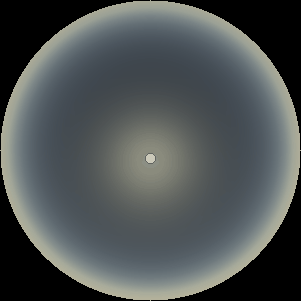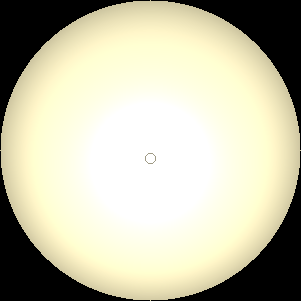Mars' dusty atmosphere Titan's hazy atmosphere Uranus' atmosphere (at 1 bar)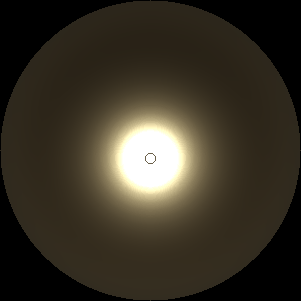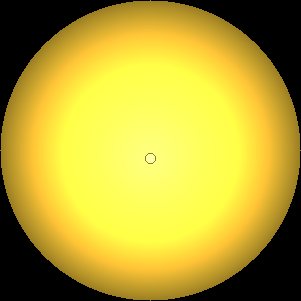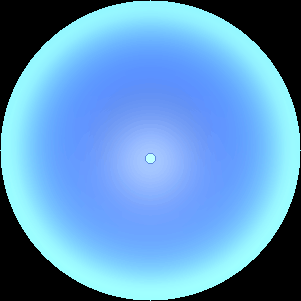Atmospheric scattering: As the Sun moves across a planetary atmosphere, its photons are scattered in different directions with a pattern defined by the wavelength of the photons. The slices top/bottom panels show the sunset for zenith angles (70,75,80,85,90) at a solar azimuth angle of +/-20 and for observational zenith angles below 45 degrees. The middle animations show all-sky views for different solar zenith angles. As it is shown for Earth’s clear atmosphere, the scattering pattern is mostly symmetric when Rayleigh dominates, while when clouds are added a non-symmetric halo is added towards the Sun direction because of Mie aerosols scattering. In the case of Mars, the atmosphere switches from a ‘brownish’ color to a ‘blueish’ tint at high solar zenith angles due to the preferential Mie scatter of Mars dust particles at red wavelengths.

Converting aerosol abundances: PSG ingests aerosol abundances X in [kg/kg] or [g/g], yet many references report aerosols in several different units. A common unit is N [particles/cm3], to determine X [kg/kg] for N=1 [particles/cm3], P=5e-3 [bar], T=340 [K], matm=18 [g/mol], particle radius (not diameter) rhaze=1e-5 [cm], ρhaze=1.36 [g/cm3]:

Vhaze = π (4/3) ⋅ rhaze3 = 4.2e-15 [cm3] Volume of each haze particle
Mhaze = Vhaze ⋅ ρhaze = 5.7e-15 [g]
Dhaze = 1E-3 ⋅ N ⋅ Mhaze = 5.7e-18 [kg/m3] (aerosol mass density)

ρatm = 1E-1 ⋅ P / (KB [1.38064852e-23] ⋅ T) = 1.065e+17 [molecules/cm3]
Xhaze = N ⋅ Mhaze ⋅ AVOG[6.022140857e23] / (ρatm ⋅ matm) = 1.8E-9 [kg/kg] (aerosol mass abundance)

 Aerosol### Defining the surface

As light arrives to a surface at a particular wavelength, it can be either be absorbed or scattered. Processes such as surface fluorescence or Raman will transfer some of this energy to a different wavelength, but for our treatment in PSG, we simply consider this as an absorption process at this wavelength. The direction and intensity of the scattered light requires of complex modeling, and several methods exist (e.g., Lambert, Hapke). The light absorbed will heat the surface, and this together with other internal sources of heat will lead to thermal emission (with an associated directionality and effectiveness/emissivity). How effective the surface scatters light is defined by the single scattering albedo, where 0 means the light is totally absorbed and to 1 the light is totally scattered back.

What is being observed or “reflected” back will depend on how this surface scatters back, and we would then require information about the observing geometry, the directability of the emissions and the geometry of the incidence fluxes. Three angles are used to define the geometry: i “incidence angle” is the angle between the Sun (or host-star) and the line perpendicular to the surface at the point of incidence, called the normal; e “emission angle” is the angle between the surface normal and the observer; and g “phase angle”, which is the angle between the source and observer (not to be confused with solar azimuth angle, which is the projection of the phase angle).

The quantity that captures how much light is being reflected towards the observer is called r(i,e,g) “bidirectional reflectance”, which is in units of per [sr], with steradians [sr] being a unit of solid angle. A common alternative quantity is the BRDF or “bidirectional-reflectance distribution function”, which describes the reflectivity of the surface with respect to a Lambertian sphere, and it is simply r/cos(i). Similarly for emission, directional emissivity is the ratio of the thermal radiance emerging at emission angle e from the surface with temperature T with respect to a black body at the same temperature.

Once the geometry (i,e,g) and the specific scattering properties (e.g., ) are defined, we would then need a scattering model to accurately model the emissions from a sphere. In PSG, four core models are available: Lambert (isotropic scattering), Hapke (parametric surface scattering), Lommel-Seeliger (weakly scattering / diffuse surfaces) and Cox-Munk (specular glint scattering model).

 Surface modeling report and details of parameters/equations Developed by Villanueva, Liuzzi, Faggi, Protopapa, May/2021Download

### Surface materials (29567 components)

Properly modeling spectroscopic features of planetary surfaces over a wide wavelength range requires a comprehensive and inclusive spectroscopic database. These parameters are used to establish the "boundary" conditions for the radiative transfer calculations. There is currently no single repository that integrates optical constants and reflectances of solid surfaces and ices over a wide spectral range (only specialized databases exist). We have identified eleven spectral databases that are applicable to the synthesis of planetary spectra, and we have developed a program to standardize and homogenize these libraries of spectral constants.

 Component### Retrieval of planetary parameters

PSG allows to compare user-provided data to synthetically generated spectra, and to perform physical retrievals of the parameters included in the model employing the optimal estimation method (Rodgers 2000). The retrieval module also implements an additional regularization parameter that improves the retrieval accuracy and convergence speed of the inverse scheme. The optimal estimator that PSG uses to get an estimate of the parameters from observations is obtained by considering a linear Gauss–Newton iterative scheme. The formal retrieval equation implemented follows this scheme:

x (γSa-1  +  KTSy-1K)  =  y (KTSy-1)

The scheme is based on the a-priori information available for the variability of the input quantities and the knowledge of the measurement uncertainty; if no error is provided, PSG will assume a 5% uncertainty. The minimum/maximum limits entered in the form for each parameter as assumed to be 5-sigma, and therefore the "variance" of each parameter is defined as (maximum-minimum)/10. Both covariance matrices, data and parameters, are assumed to be diagonal and filled with the squares of the data and parameter variances, respectively. The Jacobians are computed with forward steps of 0.1% of the maximum value.

The parameter γ is the extra regularization parameter, and acts as a tradeoff between the a-priori values given to the parameters to be retrieved (x-space) and the observations (y-space). Large values of γ will constrain the retrieval scheme more to the a-priori parameter values, hence a large γ might be recommended in case of reliable a-priori knowledge of retrieval parameters. As γ approaches 0, the solution scheme tends to a constrained least-square. For γ = 1, the Rodgers’ classical scheme is run.

Together with the optimal estimate for the parameters, the retrieval module implemented in PSG computes a series of a-posteriori quantities. The uncertainties of the parameters are computed from the a-posteriori covariance matrix, which is directly derived from the Rodgers’ formalism. In addition, the averaging kernels matrix (AK) is computed for each of the retrieved parameters. AKs provide information about the information content of the data with respect to the retrieved parameters. The diagonal of the AK matrix contains values between 0 and 1, which indicate the sensitivity of the retrieval to each parameter. Low values could be indicative of intrinsic poor sensitivity of the data themselves to the retrieval parameters, or could be due to very stringent constraints imposed to the variability of the parameters to be retrieved. In general, the contribution functions matrix and the AKs are presented to highlight the properties of the retrieved parameters, and their significance. AKs can also be provided prior to the retrieval, to allow the user to evaluate the information content of the spectrum, and tune the input parameters. The retrieval provides also an accurate a-posteriori estimation of the measurement uncertainty.

Beyond fitting the data to the spectroscopic model, PSG can also correct for a broad range of instrumental issues typically affecting data (see the figure below). By employing pre-computed telluric spectra, PSG can also fit the water and column parameters affecting ground-based data. For fitting these instrumental effects, PSG employs the Levenberg–Marquardt algorithm, also known as the damped least-squares method, in solving the non-linear least squares problem. This method interpolates between the Gauss–Newton algorithm and the method of gradient descent, with the Levenberg–Marquardt method being more robust, meaning that it finds a solution even if the initial conditions are far from the final solution. The Levenberg-Marquardt algorithm is based on the MPFIT program (Craig Markwardt), which draws from the robust package called MINPACK-1 (Garbow, Hillstrom and More).

 Optimal Estimation Method (OEM) C/C++ package Developed by Liuzzi and Villanueva, Sep/2018 Based on methodologies described in Rodgers 2000 and therein.Download Levenberg-Marquardt (LM) minimization C/C++ package Developed by Craig Markwardt, June/2016 Based on the MINPACK-1 (Garbow, Hillstrom and More).Download

The input file should be formatted as a text file with two or three columns. The first column should indicate the frequency/wavelength of each pixel, while the second column should describe the measured flux. A third column (optional) should indicate the 1-sigma uncertainty in flux, and if no error is provided, PSG will assume a 5% uncertainty. See below a list of example configuration files (that also include the data):
• Comet IR retrieval: retrieval of production rates and rotational temperatures in comet C/2007 W1 (Boattini) from high-resolution Keck/NIRSPEC data acquired on July/9/2008 (Villanueva et al. 2011, Icarus 216, 227-240).
• Mars retrieval: retrieval of deuterated water from high-resolution Keck/NIRSPEC data acquired in January/2014. This is a relatively complex and expensive retrieval, in which several instrumental issues are removed simultaneously (Villanueva et al. 2015, Science 348-6231).
• Comet radio retrieval: retrieval of cometary methanol production rate and its rotational temperature from high-resolution data of comet C/2002 T7 (LINEAR) acquired with the Heinrich Hertz Submillimeter Telescope (Villanueva et al. 2005, AAS/DPS meeting).
• Earth retrieval: retrieval of methane and carbon dioxide in Earth's atmosphere via laser heterodyne radiometry (LHR). This is part of a ground-based network of stations located across the planet (Wilson et al. 2017).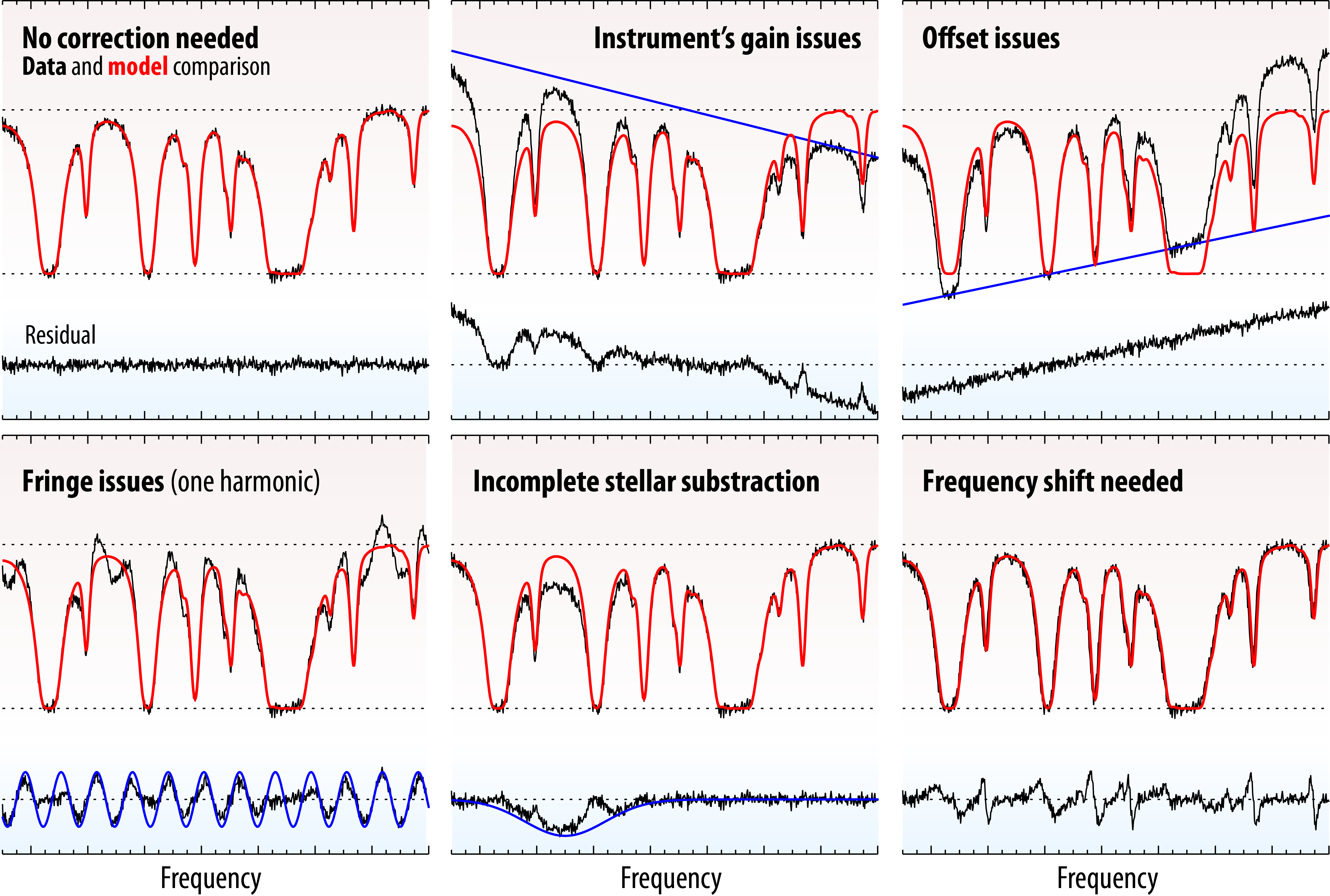Data corrections: beyond performing the retrieval of planetary parameters, PSG can also correct for several typical issues affecting spectroscopic data. The different panels show how these issues could affect the data, and their impact on the residuals. The methods employed to remove these instrumental effects are described in Villanueva et al. 2013 (Icarus 223, 11–27).